# An airplane

An airplane flies 1440 km in 2 1/4 hours. What is its average speed in km per hour?

v =  640 km/h

### Step-by-step explanation: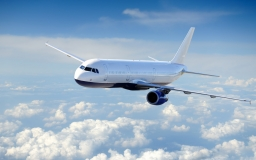Did you find an error or inaccuracy? Feel free to write us. Thank you!Tips to related online calculators
Need help to calculate sum, simplify or multiply fractions? Try our fraction calculator.
Do you want to convert velocity (speed) units?
Do you want to convert time units like minutes to seconds?

## Related math problems and questions:

• A bus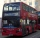A bus is scheduled to travel a distance 121 km in 2 3/4 hours. What average rate of speed must be maintained to arrive on schedule?
• Two airportsTwo airports are 2690 km away. From the first airport, the airplane flies at a speed of 600 km/h, from a second airplane at a speed of 780 km/h. When will they meet if they flew at 10:00? How far from the first airport?
• Motorcyclist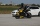From Trutnov, the motorcyclist started at an average speed of 60km/hour. At 12.30hrs the passenger car was started at a speed of 80km/hour. How many hours and at what distance from Trutnov will car catch a motorcycle?
• Average speed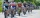The average speed of a pedestrian who walked 10 km was 5km/h, the average speed of a cyclist on the same track was 20km/h. In how many minutes did the route take more than a cyclist? Q
• Tourist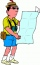A tourist walked an average speed of 3.5 km/h route in 6 hours. Calculate how many hours he would have passed at an average speed of 5.5 km/h.
• Two cars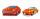From the town A to town B started two cars. The first at 7:00 at average speed 60 km per hour, the second at 10:00 at average speed 100 km per hour. The first car will not stay in B, and on the way back meet the second car at half way from A to B. At what
• Average speed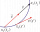The car drove on one section of the highway for half an hour at a speed of 80 km/h. Then he reduced his speed to 60km/h. It went three-quarters of an hour at a speed of 60 km/h. What was the average speed of the car?
• Two aircraftTwo planes fly to the airport. At some point, the first airplane is away from the airport 98 km and the second 138 km. The first aircraft flies at an average speed of 420 km/h, the second average speed is 360 km/h, while the tracks of both planes are perp
• Day trip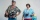A group of tourists went on a day trip. For two hours they walked at a speed of 6 km/h, for another hour they climbed a steep hill at a speed of 2 km/h, spent an hour and a half at lunch, then in an hour they inspected the castle garden at a free step at
• Average speed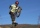The average speed of a good cyclist is 30 km/h. The average speed of the less able is 20 km/h. They both set off on the same route at the same time. Good cyclist drove it 2 hours earlier. How long was the route?
• Two portsFrom port A on the river, the steamer started at an average speed of 12 km/h towards port B. Two hours later, another steamer departed from A at an average speed of 20 km/h. Both ships arrived in B at the same time. What is the distance between ports A an
• Sputnik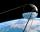The first Earth satellite was flying at speed 8000 m/s. At that rate he circled the earth in 82 minutes. Jet flies at an average speed 800 km/h. How long would it take circle the earth round?
• Aircraft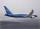If an airplane flies 846 km/h for 8 h, how far does it travel?
• The convoy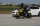During a military exercise, the convoy left the camp at 5 hours at a speed of 40 km/h. Half an hour later, a motorcycle clutch was sent at a speed of 70 km/h. How long before the motorcycle clutch reaches the column?
• Average speedWhen the bus stops at a bus stop driving average speed is 45 km/h. If it did not stop, it would drive at a speed of 54 km/h. How many minutes of every hour does it spend at stops?
• Car driver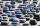The car driver is in town A and is scheduled to be in town B at a specified hour. If it travels at an average speed of 50km/h, it will arrive in city B 30 minutes later. But if he travels at an average speed of 70km/h, he would arrive half an hour earlier
• MovementFrom the crossing of two perpendicular roads started two cyclists (each at the different road). One runs at average speed 28 km/h, the second at average speed 24 km/h. Determine the distance between them after 45 minutes cycling.